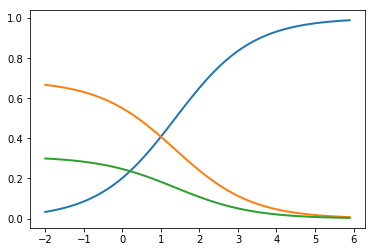To be a great engineer.

0%

Softmax函数公式如下，其中yi代表分类i的评分:

$$softmax(y_{i})=\frac{\exp y_{i}}{\sum_{j} \exp y_{j}}$$def softmax(x):
return np.exp(x)/np.sum(np.exp(x),axis=0)

P.S.可以使用以下代码，复现上图的结果

import matplotlib.pyplot as plt
x = np.arange(-2, 4 , 0.1)
scores = np.vstack([x, 0.4 * x , 0.2 * x])

plt.plot(x, softmax(scores).T, linewidth=2)
plt.show()